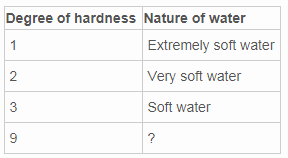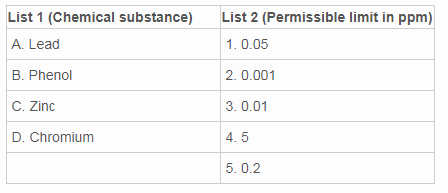# Test: Chemical Characteristics Of Water

## 10 Questions MCQ Test Environmental Engineering | Test: Chemical Characteristics Of Water

Description
Attempt Test: Chemical Characteristics Of Water | 10 questions in 25 minutes | Mock test for Chemical Engineering preparation | Free important questions MCQ to study Environmental Engineering for Chemical Engineering Exam | Download free PDF with solutions
QUESTION: 1

### Chlorides are estimated by titration with a standard silver nitrate solution by using _______as indicator.

Solution:

Explanation: 2-3 drops of potassium chromate are added to appropriate amount of water sample and then it is titrated with a standard solution of silver nitrate.

QUESTION: 2

### State whether the following statement is True or False. Carbonate hardness can be removed by adding lime to water.

Solution:

Explanation: Carbonate hardness is due to the presence of carbonates and bicarbonates of calcium and magnesium in water and can be removed by boiling or by adding lime to it.

QUESTION: 3

### Which of the following statement is wrong regarding permanent hardness?

Solution:

Explanation: Permanent hardness is called non-carbonate hardness whereas temporary hardness is called carbonate hardness.

QUESTION: 4

One degree of hardness is equivalent to ___ ppm

Solution:

Explanation: Water is said to have one degree of hardness when its soap destroying power is equivalent to the effect of 14.25mg of calcium carbonate in one litre of water.

QUESTION: 5What can be filled in place of the question mark in the above table?

Solution:

Explanation: When the degree of hardness is 9, then it indicates the hard water. For excessive hard water, it is 15 and for very hard water, it is 11.

QUESTION: 6

In which method of determining total hardness of water is based on the premise that hardness producing substance react with soap and form insoluble compounds before lather is produced?

Solution:

Explanation: In Clark’s method, total hardness is found by determining the standard soap solution required to obtain permanent lather with the water sample of known volume.

QUESTION: 7

What is the indicator used in EDTA method?

Solution:

Explanation: Hardness is determined by using Ethylene diamine tetra-acetic acid using Erio chrome, black T as indicator at a pH between 8.5 and 11.

QUESTION: 8

The permissible limit of pH preferred for potable water is ___ppm.

Solution:

The permissible limit of pH for potable water should not exceed 9 and should not be less than 6.5 and the preferred limit is 7-8.5.

QUESTION: 9

Given list 1 and list 2, find which of the following pair is correctly matched?Solution:

Explanation: Lead has a permissible limit of 0.05ppm, phenol has 0.001ppm, zinc has 5ppm and chromium has a permissible limit of 0.05ppm in water.

QUESTION: 10

What is the concentration of H+ ions in moles/L in water if the pOH value is 6?

Solution:

Explanation: pH + pOH = 14
pOH = 6, so pH = 14-6= 8
H+ = 10-pH = 10-8moles/L.Use Code STAYHOME200 and get INR 200 additional OFF Use Coupon Code